# Quick intro to the knotty subject

This is a brief, rough, sketch of the workshops I conducted for gifted high-school students in Lublin, Poland, Dec 2022.

## Intro

No doubt: we all had to untie some annoyingly tangled knot at some point. So here's a fun problem: Is there any way to rigorously tell if one knot is more difficult to be untangled than another? Or even if two unlike looking knots are the same or actually different? You might be tempted to think that in practice it is easy to tell. Then consider the (un)knots in Fig. 1 and Fig. 2: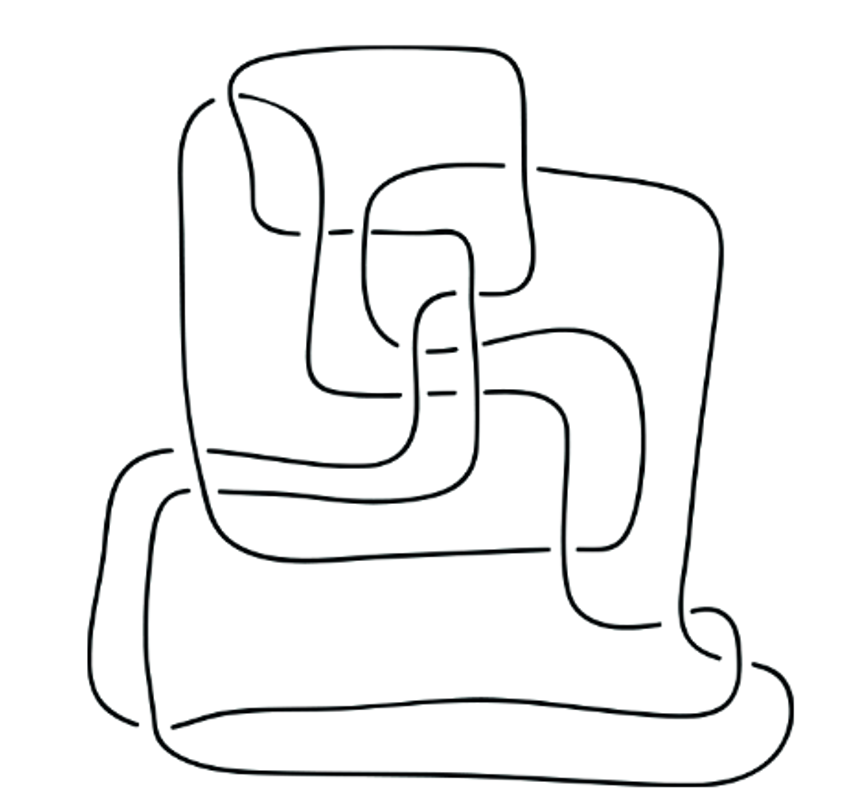What is plotted above are 2D projections (known as knot diagrams) of the simple loop (living in 3D), just twisted in some elaborate ways. Curious! Okay, since it might not be so easy to tell if two knot diagrams correspond to the same or different knot. At least, is there any algorithm, which, if one followed it rigorously, would guarantee that in finite number of steps we would get the simplest possible form of the knot? Short answer: yes. We will explore it in the next section.

## Reideimeister moves

To understand the algorithmic approach, we will have to clarify few things.

First, we need to say what techniques of disentangling knots are allowed. You might be sneaky, and just like Alexander the Great (at least in one version of the legend) start cutting the knot under consideration. To the disappointment of Zeus, this is not allowed: we deal with a beautiful branch of math known as topology. In topology, we are already allowed to do a lot of things (e.g., we can deform the precise geometrical shape of the knot in any way we want!), but cutting/glueing stuff is not one of it, period.

Second, what does "the simplest possible form of the knot" mean? Simplest possible knot corresponds to the alternating knot. An alternating knot has the following property: if one followed the thread on the knot diagram, she would see over-crossing, followed by under-crossing, followed by over-crossing, followed by ... - you get the "alternating" pattern. See Fig below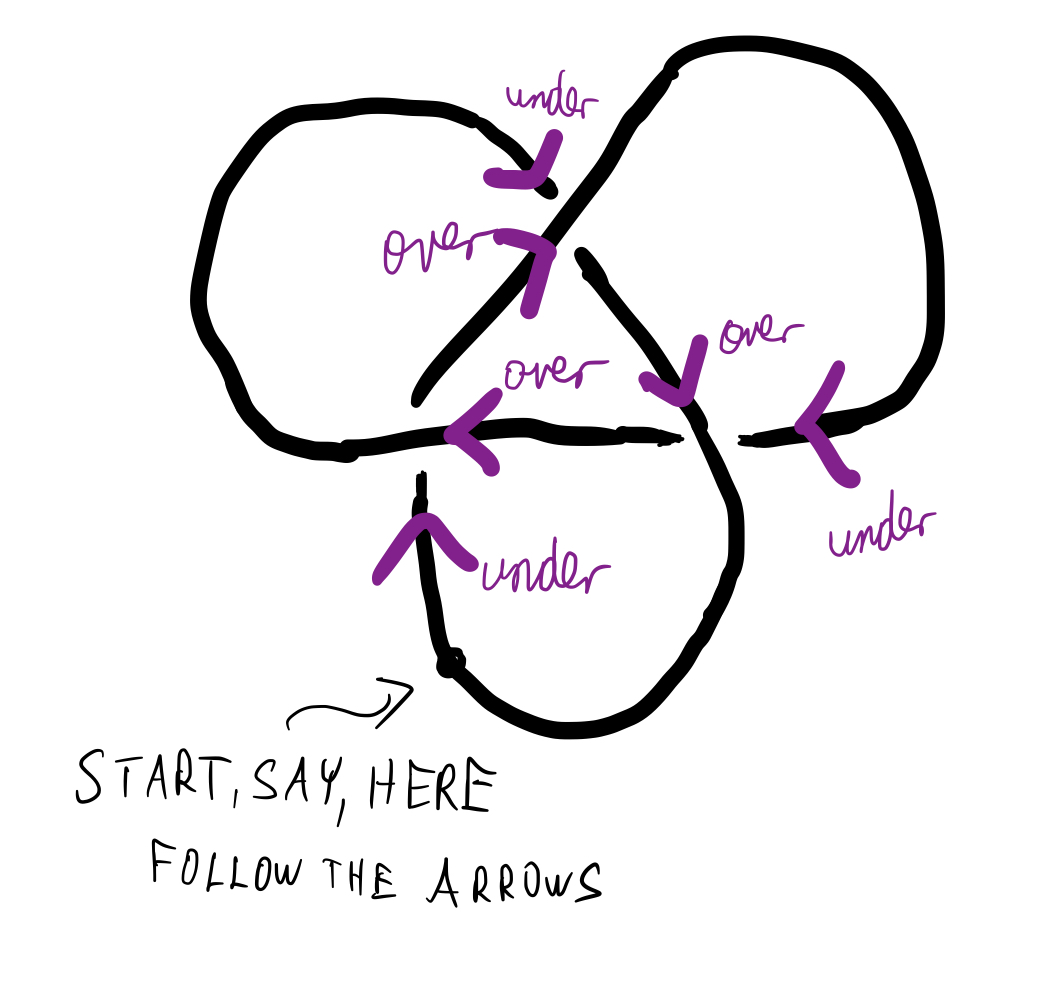Exercise: Convince yourself that alternating knot cannot be further simplified. Can you think about any exceptions to this? (Hint: think about a loop with a single twist)

Equipped with these two points we can proceed to the algorithm. The algorithm consists of iterative application of three basic disentangling moves, known as Reideimeister moves, and presented in Fig. 4 below: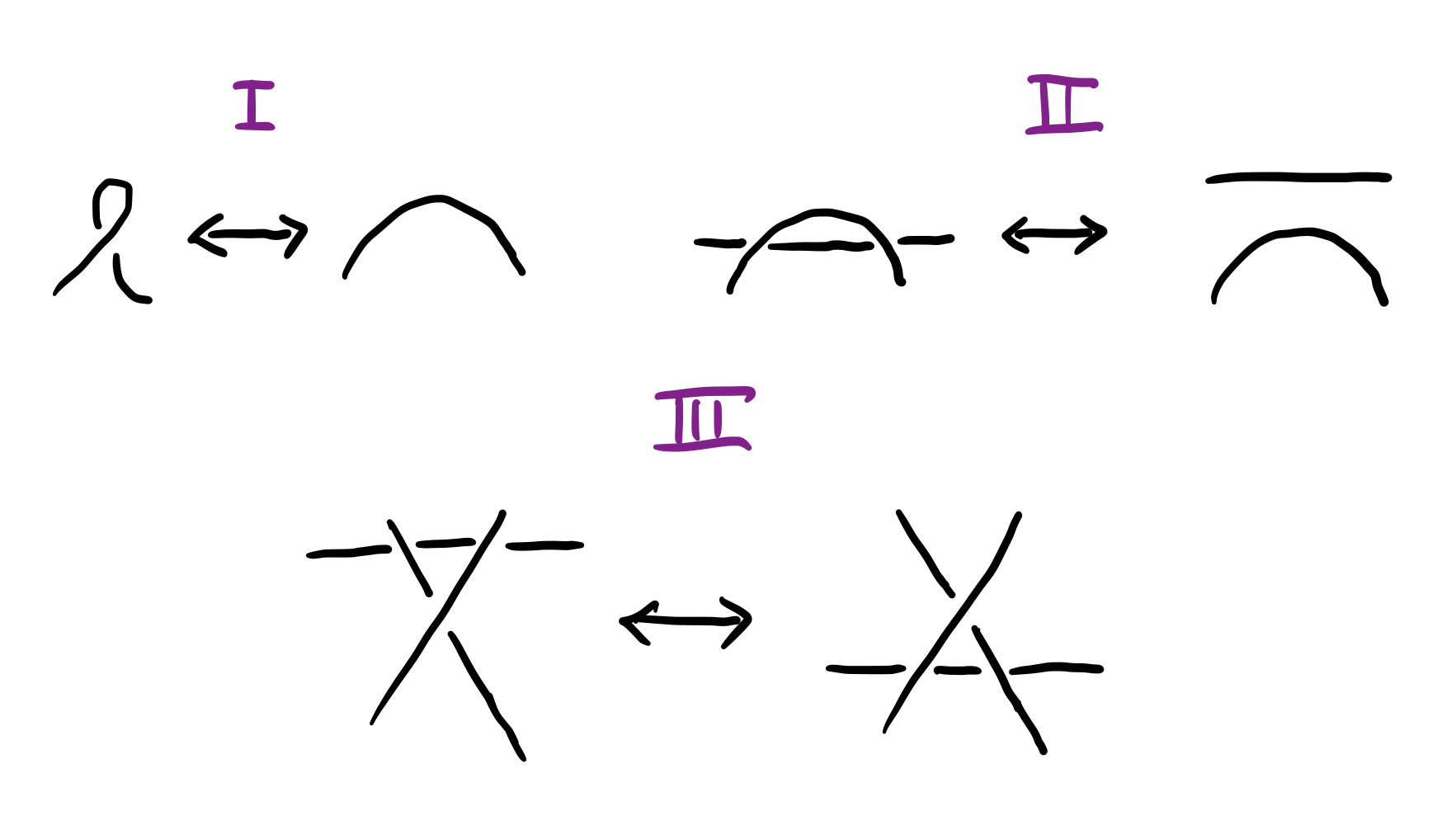Simple! We will demonstrate the R-moves by disentangling the knot below (which corresponds to trefoil knot in Fig. 3 with one crossing changed from overcrossing to undercrossing):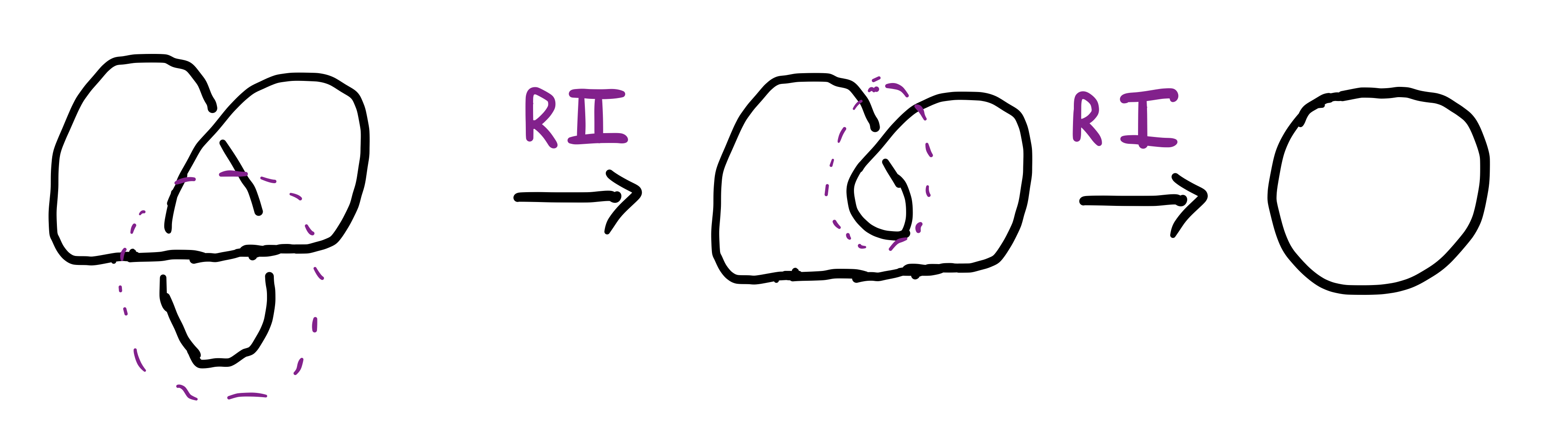In the above case it turned out that the original knot was in fact equivalent to the simple loop (unknot). Okay, great! To test your understanding solve the following exercise:

Exercise: Simplify the knot diagram in Fig. 6 as much as possible using three Reidemeister moves.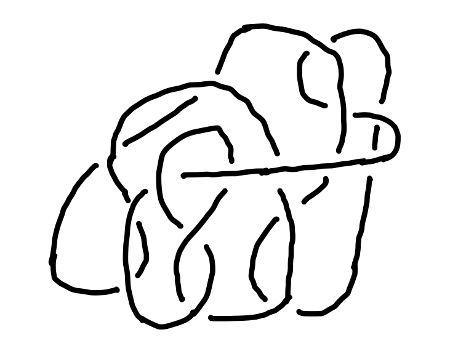## Knot invariants

We have repeatadely seen that by Reidemeister moves we can convert knot diagrams which initially do not look alike might in fact correspond to the same knot. It of course means that each knot might have many different diagrams which are related by a series of R-moves (or in this context we might also loosely say "topologically equivalent"). It would be nice if to each knot diagram we might have associated a number (or set of numbers) which would be neccesarily the same for two knot diagrams corresponding to the same knot. This is the idea of a knot invariant and restated in the Fig. 7 below: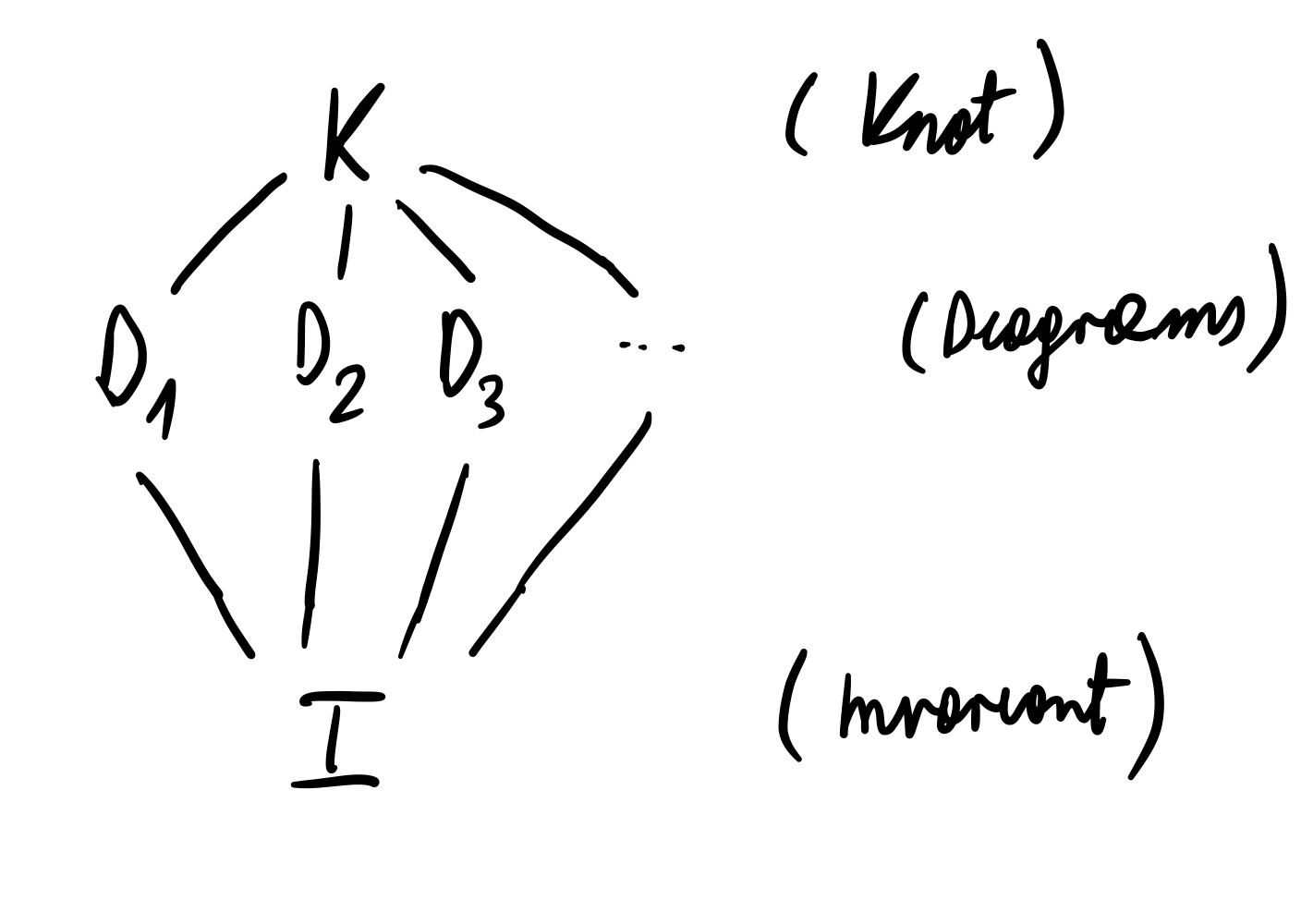What are some examples of knot invariants? Here I will just list some of them: unknotting number, Jones polynomial, X-polynomial, fundamental group of the knot, existence of knot tri-coloring etc. To any knot we might associate multiple knot invariants. We will see that, sometimes, a particular invariant for two different knots might return the same value.

Perhaps the simplest invariant to describe is the unknotting number. Unknotting number is the minimum number of changes of overcrossings to undercrossings (or vice versa) one would have to perform to get a knot equivalent (up to R-moves) to a simple loop (unknot). We have seen before in Fig. 5 that trefoil knot has an unknotting number 1. To train the unknotting number calculation consider the following exercise:

Exercise: Calculate the unknotting number of the knots in Fig. 8.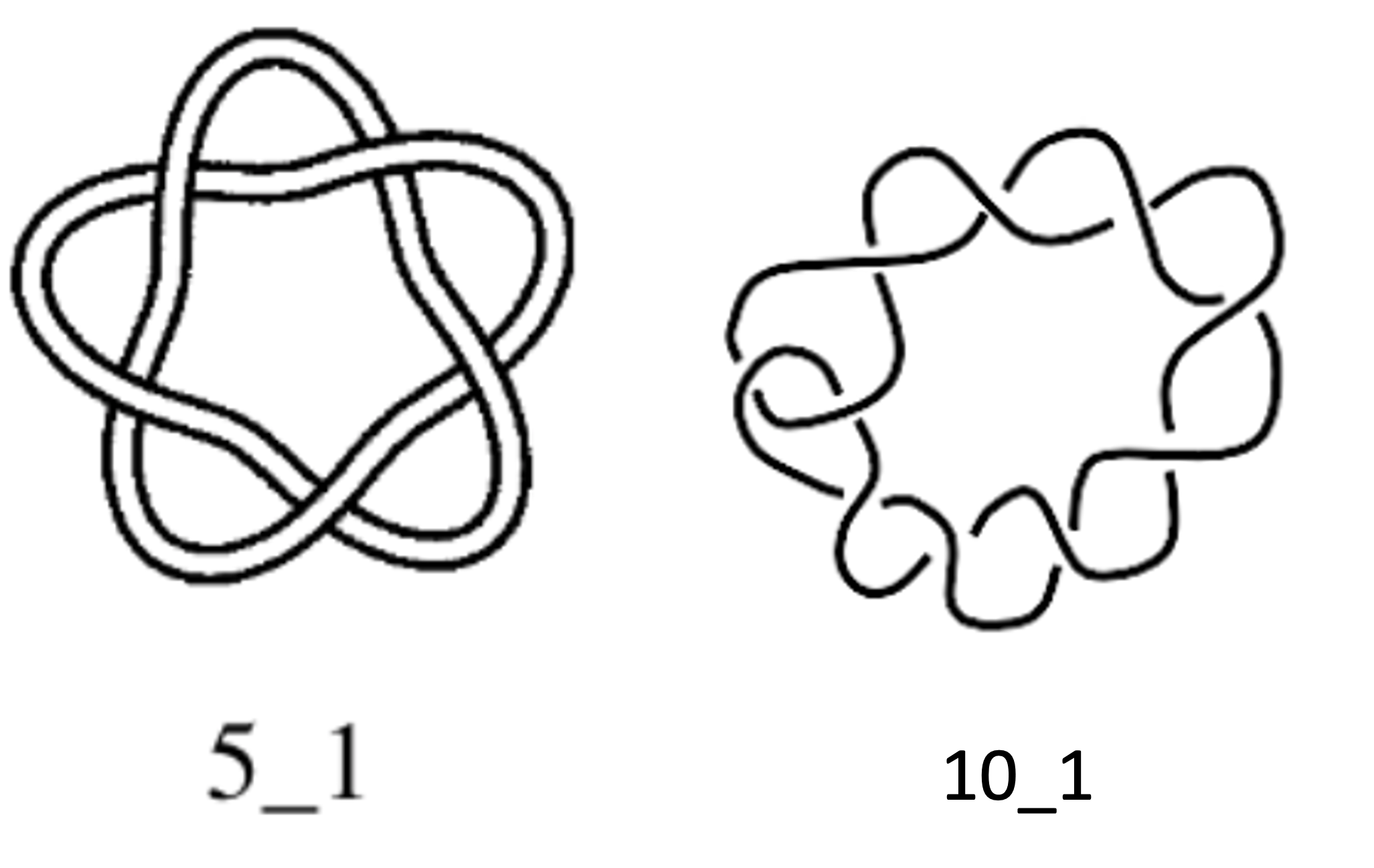## Conclusion

Alright, we have studied how to convert one equivalent knot diagram into another such that we can create simplest-looking knot diagram (use Reidemeister moves). We have also discussed idea of knot invariants and learnt how to calculate one of them (unknotting number). If you would like to learn more about mathematical basics or applications of knot theory to other fields (such as DNA biology or quantum computing) see further reading below.